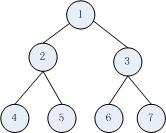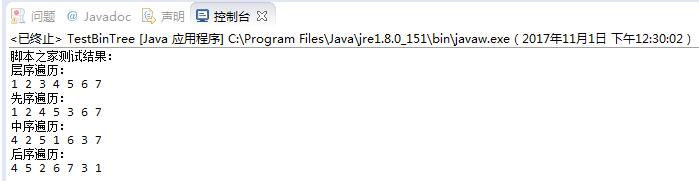# Java完全二叉树的创建与四种遍历方法分析```import java.util.ArrayDeque;
import java.util.ArrayList;
import java.util.List;
import java.util.Queue;
/**
* 定义二叉树节点元素
* @author bubble
*
*/
class Node {
public Node leftchild;
public Node rightchild;
public int data;
public Node(int data) {
this.data = data;
}
}
public class TestBinTree {
/**
* 将一个arry数组构建成一个完全二叉树
* @param arr 需要构建的数组
* @return 二叉树的根节点
*/
public Node initBinTree(int[] arr) {
if(arr.length == 1) {
return new Node(arr);
}
List<Node> nodeList = new ArrayList<>();
for(int i = 0; i < arr.length; i++) {
}
int temp = 0;
while(temp <= (arr.length - 2) / 2) { //注意这里，数组的下标是从零开始的
if(2 * temp + 1 < arr.length)
nodeList.get(temp).leftchild = nodeList.get(2 * temp + 1);
if(2 * temp + 2 < arr.length)
nodeList.get(temp).rightchild = nodeList.get(2 * temp + 2);
temp++;
}
return nodeList.get(0);
}
/**
* 层序遍历二叉树
* @param root 二叉树根节点
* @param nodeQueue ，用到的队列数据结构
*/
public void trivalBinTree(Node root, Queue<Node> nodeQueue) {
Node temp = null;
while ((temp = nodeQueue.poll()) != null) {
System.out.print(temp.data + " ");
if (temp.leftchild != null) {
}
if (temp.rightchild != null) {
}
}
}
/**
* 先序遍历
* @param root 二叉树根节点
*/
public void preTrival(Node root) {
if(root == null) {
return;
}
System.out.print(root.data + " ");
preTrival(root.leftchild);
preTrival(root.rightchild);
}
/**
* 中序遍历
* @param root 二叉树根节点
*/
public void midTrival(Node root) {
if(root == null) {
return;
}
midTrival(root.leftchild);
System.out.print(root.data + " ");
midTrival(root.rightchild);
}
/**
* 后序遍历
* @param root 二叉树根节点
*/
public void afterTrival(Node root) {
if(root == null) {
return;
}
afterTrival(root.leftchild);
afterTrival(root.rightchild);
System.out.print(root.data + " ");
}
public static void main(String[] args) {
TestBinTree btree = new TestBinTree();
int[] arr = new int[] {1,2,3,4,5,6,7};
Node root = btree.initBinTree(arr);
Queue<Node> nodeQueue = new ArrayDeque<>();
System.out.println("测试结果：");
System.out.println("层序遍历：");
btree.trivalBinTree(root, nodeQueue);
System.out.println("\n先序遍历：");
btree.preTrival(root);
System.out.println("\n中序遍历：");
btree.midTrival(root);
System.out.println("\n后序遍历：");
btree.afterTrival(root);
}
}

```①若k=1，则该结点为根结点，它没有父结点；若k>1，则该结点的父结点编号为INT（k/2）。

②若2k≤n，则编号为k的结点的左子结点编号为2k；否则该结点无左子结点（显然也没有右子结点）。

③若2k+1≤n，则编号为k的结点的右子结点编号为2k+1；否则该结点无右子结点。